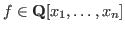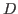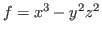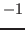### Computation of annihilating ideals by kan/sm1 and ox_asir

Letbe the ring of differential operators. For a given polynomial, the annihilating ideal ofis defined asHere,denotes the action ofto functions. The annihilating ideal can be regarded as the maximal differential equations for the function. An algorithm to determine generators of the annihilating ideal was given by Oaku (see, e.g., [16, 5.3]). His algorithm reduces the problem to computations of Gröbner bases inand to find the minimal integral root of a polynomial. This algorithm (the function annfs) is implemented by kan/sm1 , for Gröbner basis computation in, and ox_asir, to factorize polynomials to find the integral roots. These two OpenXM compliant systems are integrated by the OpenXM protocol.

For example, the following is a sm1 session to find the annihilating ideal for.

sm1>[(x^3-y^2 z^2) (x,y,z)] annfs ::
Starting ox_asir server.
Byte order for control process is network byte order.
Byte order for engine process is network byte order.
[[-y*Dy+z*Dz, 2*x*Dx+3*y*Dy+6, -2*y*z^2*Dx-3*x^2*Dy,
-2*y^2*z*Dx-3*x^2*Dz, -2*z^3*Dx*Dz-3*x^2*Dy^2-2*z^2*Dx],
[-1,-139968*s^7-1119744*s^6-3802464*s^5-7107264*s^4
-7898796*s^3-5220720*s^2-1900500*s-294000]]

The last polynomial is factored asand the minimal integral root isas shown in the output.

Similarly, an algorithm to stratify singularity  is implemented by kan/sm1 , for Gröbner basis computation in, and ox_asir, for primary ideal decompositions.

Nobuki Takayama 2017-03-30